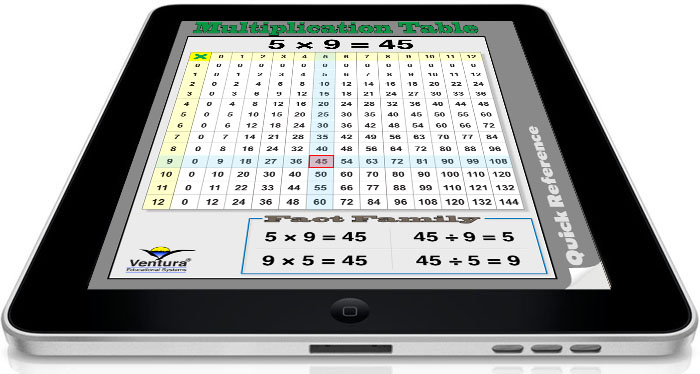A Quick Reference Tool for Elementary Math Students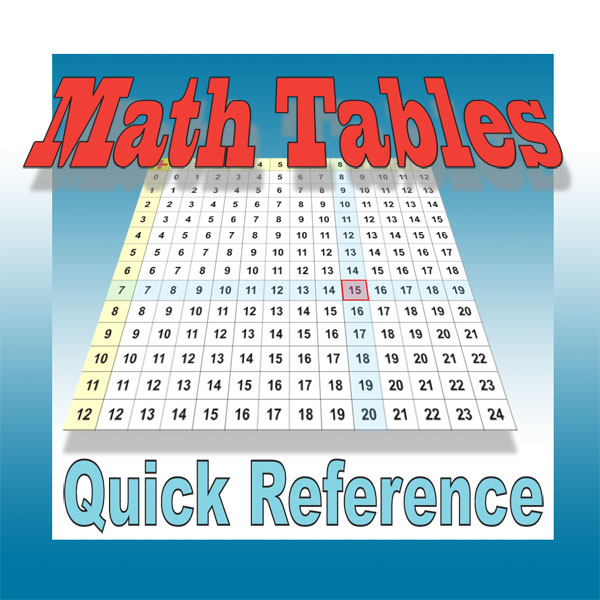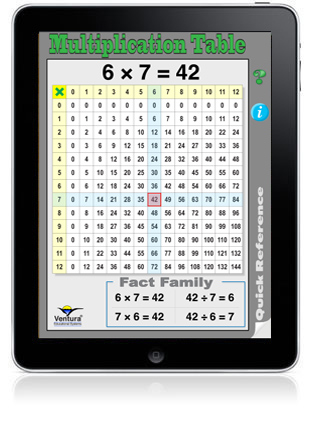Check out our Hands-On Math apps for a comprehensive tools for teaching elementary math! Most adults will remember the folders that they carried to school with a times table chart. Well today's kids are carrying iPads. Math Tables Quick Reference provides interactive access to addition and multiplication tables. Math Tables Quick Reference is a basic information tool for elementary math students. It provides a simple way to access and review the addition tables, multiplication tables and fact families. Here are a few of the ways you will use Math Tables Quick Reference everyday with your students: • Use Math Tables Quick Reference as an informational tool to help students understand and review the relationships and patterns of the addition and multiplication tables. • Use Math Tables Quick Reference as a quiz to help students practice addition and multiplication facts and to learn about Fact Families. •Use simple gestures to select an addition or multiplication fact and instantly see the related Fact Family. •Math Tables Quick Reference is perfect for every elementary age math student.
 An Interactive Quick Reference Tool Drag your finger across the screen to move the marker and define a math fact. Instantly see the related fact and the fact family for any of the table's columns and rows. Topics include: Addition Facts Multiplication Facts Subtraction Facts Division Facts Fact Families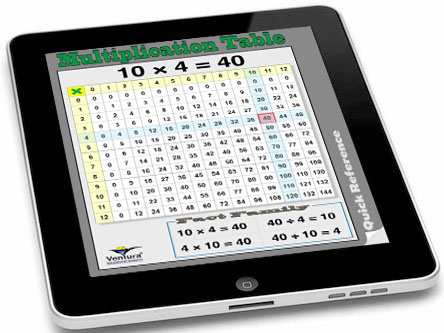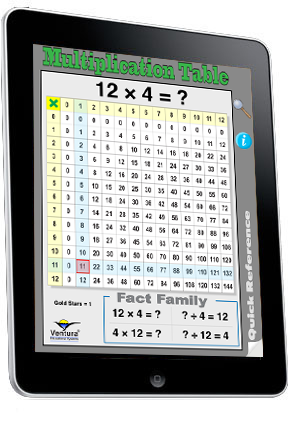Addition and Multiplication Quizzes Tap the question mark icon to activate the quiz. Problems are randomly generated using the numbers from 0 to 12. The student drags the marker to indicate an answer. A gold star appears when the correct answer is selected. Tapping the gold star generates the next problem. Problems for either addition or multiplication are presented. The interface is designed so that students can find the correct answer. Correct answers are rewarded with a gold star. Teachers and parents may want to challenge students to earn ten gold stars.
 \$0.99